﻿ 基于光纤网的光缆线路温度突变定位方法研究 Research on Temperature Mutation Location Method of Optical Cable Line Based on Optical Fiber Network

Smart Grid
Vol.08 No.04(2018), Article ID:26453,8 pages
10.12677/SG.2018.84037

Research on Temperature Mutation Location Method of Optical Cable Line Based on Optical Fiber Network

Shudong Li1, Hongjie Zhu1, He Gong1, Xueyun Xu1, Xiaowei Zhu1, Sheng Yang1, Jiang Zhu1, Xiaotao Chen2

1Baoshan Power Supply Bureau of Yunnan Power Grid Corporation of China Southern Power Grid, Baoshan Yunnan

2Kunming Chuangxuntong Communication Co., Ltd., Kunming Yunnan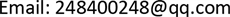Received: Jul. 21st, 2018; accepted: Aug. 4th, 2018; published: Aug. 16th, 2018ABSTRACT

The normal operation of optical fiber cable is an important condition to ensure the safe and stable operation of power system. It is important to grasp the real-time operating status of the optical fiber line in time. The Optical Time Domain Reflectometry (OTDR) is a technique for studying the quality of optical fibers. It mainly measures the power loss and reflection characteristics of optical fibers. If there are fiber faults (fiber breaks, bends, connectors, and connectors), these numbers can be greatly affected. Therefore, OTDR equipment can be used to locate fiber faults. At the same time, this paper also introduces the principle of wavelet transform to detect the noise and singularity of the signal collected by OTDR. Based on the above principles, an optical fiber fault location system is designed, which can be divided into three levels: a front-end hardware detection device, an intermediate data processing center, and an intelligent terminal installed with an optical cable fault location system.

Keywords:Optical Fiber Network, Optical Fiber Cable, Mutation, Temperature Location

1中国南方电网云南电网公司保山供电局，云南 保山

2昆明创讯通通信有限公司，云南 昆明1. 引言

2. OTDR技术研究

$P\left(x\right)=P\left(0\right)×{10}^{-\left(\alpha x/10\right)}$

${P}_{bs}\left(x\right)=P\left(x\right)\gamma \left(x\right){10}^{-\left(\alpha x/10\right)}=P\left(0\right)\gamma \left(x\right)×{10}^{-2\left(\alpha x/10\right)}$ (1)

$\gamma \left(x\right)=\left(VT/2\right){\alpha }_{R}\cdot S$ (2)

V表示光在纤芯中的群速率， ${\alpha }_{R}$ 表示瑞利散射系数，S表示背向散射功率与瑞利散射总功率之比。

${P}_{bs}\left(0\right)=P\left(0\right)\gamma \left(0\right)$ (3)

$\alpha =\frac{5}{x}\left[\mathrm{log}\frac{{P}_{bs}\left(0\right)}{{P}_{bs}\left(x\right)}-\mathrm{log}\frac{\gamma \left(0\right)}{\gamma \left(x\right)}\right]$ (4)

$\alpha =\frac{5}{x}\left[\mathrm{log}\frac{{P}_{bs}\left(0\right)}{{P}_{bs}\left(x\right)}\right]$ (5)

${P}_{f}\left(x\right)=P\left(x\right)\lambda \left(x\right){10}^{-\left(\alpha x/10\right)}=P\left(0\right)\lambda \left(x\right)×{10}^{-2\left(\alpha x/10\right)}$ (6)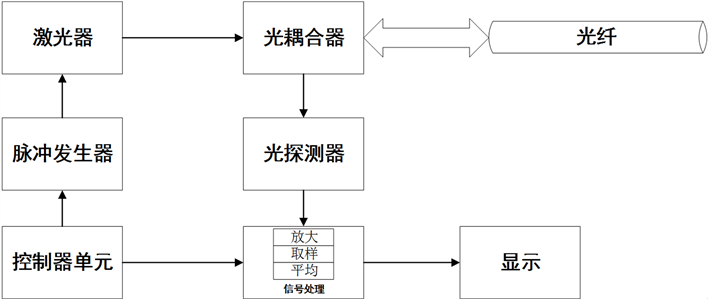Figure 1. OTDR principle

$\begin{array}{c}{P}_{i}\left(x\right)={P}_{bs}\left(x\right)+{P}_{f}\left(x\right)\\ =P\left(0\right)\gamma \left(x\right)×{10}^{-2\left(\alpha x/10\right)}+P\left(0\right)\lambda \left(x\right)×{10}^{-2\left(\alpha x/10\right)}\\ =P\left(0\right)\left(\gamma \left(x\right)+\lambda \left(x\right)\right)×{10}^{-2\left(\alpha x/10\right)}\end{array}$ (7)

$t=2L{n}_{1}/c$ (8)

3. 系统研究与设计

3.1. 光纤网架构

3.2. 传感子系统

3.3. 光缆线路温度定位系统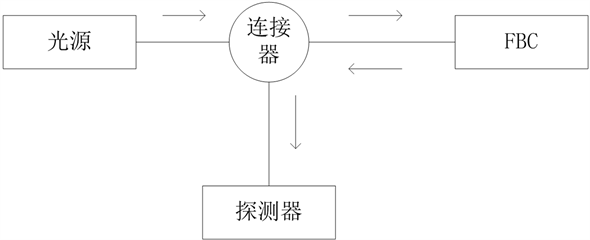Figure 2. Sensor subsystem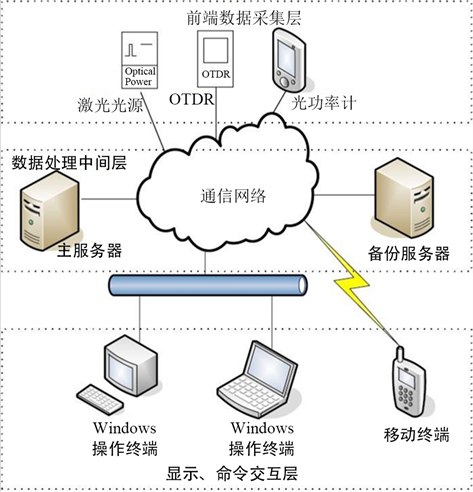Figure 3. System components and connections

4. 光缆线路温度突变监测

4.1. 突变检测算法

${\psi }_{S}\left(x\right)=\frac{1}{s}\psi \left(\frac{x}{s}\right)$ (9)

$Wf\left(s,x\right)=f\cdot {\psi }_{s}\left(x\right)$ (10)

4.2. 小波去噪

$s\left(i\right)=f\left(i\right)+\sigma \cdot e\left(i\right),i=1,2,\cdots ,n-1$ (11)

4.3. 系统工作流程

4.4. OTDR曲线分析模块的分析与设计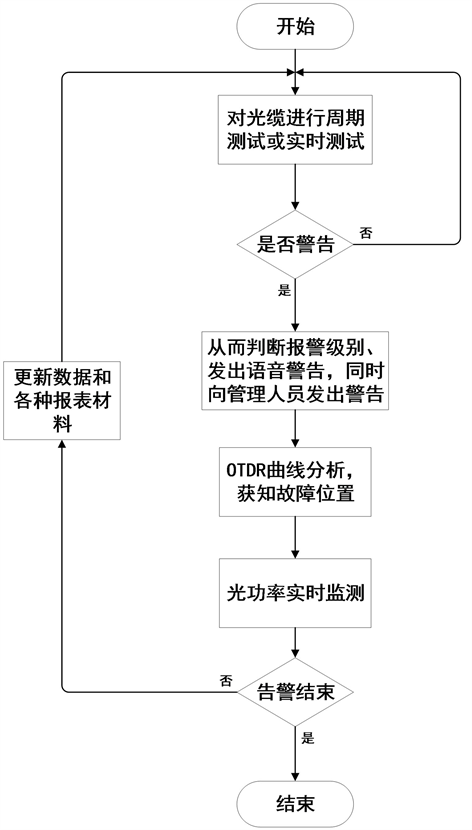Figure 4. Optical cable fault location work flow chart

5. 仿真分析

$\varphi \left(t,a\right)=\frac{1}{a}\text{e}{}^{-\frac{{t}^{2}}{2{a}^{2}}}$ (12)Figure 5. Event detection process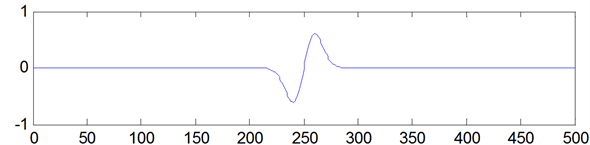Figure 6. Wavelet simulation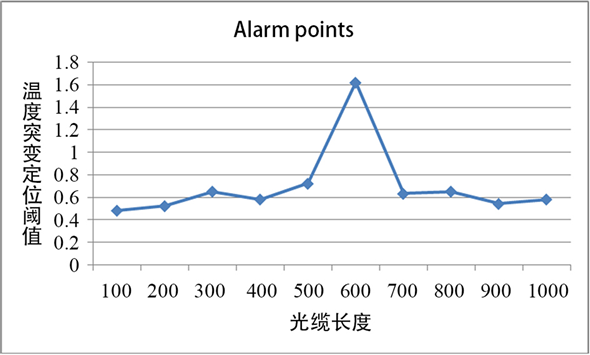Figure 7. The temperature point of the fiber optic cable

6. 结论

Research on Temperature Mutation Location Method of Optical Cable Line Based on Optical Fiber Network[J]. 智能电网, 2018, 08(04): 335-342. https://doi.org/10.12677/SG.2018.84037

1. 1. 李伟. 结合小波变换与GIS地图的电力通信光缆故障定位及管理系统[D]: [硕士学位论文]. 重庆: 重庆大学, 2015.

2. 2. 李波. 基于GIS的通信光缆故障定位技术[D]: [硕士学位论文]. 大连: 大连理工大学, 2014.

3. 3. 张静. 电力光纤故障检测及抢修最优路径方法研究[D]: [硕士学位论文]. 保定: 华北电力大学(保定), 2013.

4. 4. 涂超. 基于Google Maps的光缆故障定位方法的研究与实现[D]: [硕士学位论文]. 北京: 华北电力大学, 2012.

5. 5. 王洪亮, 曹敏, 周洁. 山地变电站气象信息光纤传感远程监测技术研究[J]. 电波科学学报, 2018, 33(2): 225-230.

6. 6. 黎华. 基于OTDR和光功率测试的光纤故障监测应用方法[J]. 计算机测量与控制, 2014, 22(5): 1357-1359.

7. 7. 卢乐书, 邓晓智. 基于改进型OTDR的光纤故障定位算法[J]. 光通信技术, 2017(11).

8. 8. 尧昱, 张静, 张乐, 等. 基于OTDR的PON光纤故障定位方法[J]. 电信科学, 2012, 28(10): 143-148.

9. 9. 潘青, 张引发, 邓大鹏, 等. 一种改进的OFDR技术在光纤故障定位中的应用[J]. 光通信技术, 2015, 39(7).

10. 10. 曹贝贝. 基于OTDR的信息采集与处理技术研究[D]: [硕士学位论文]. 北京: 北京邮电大学, 2017.